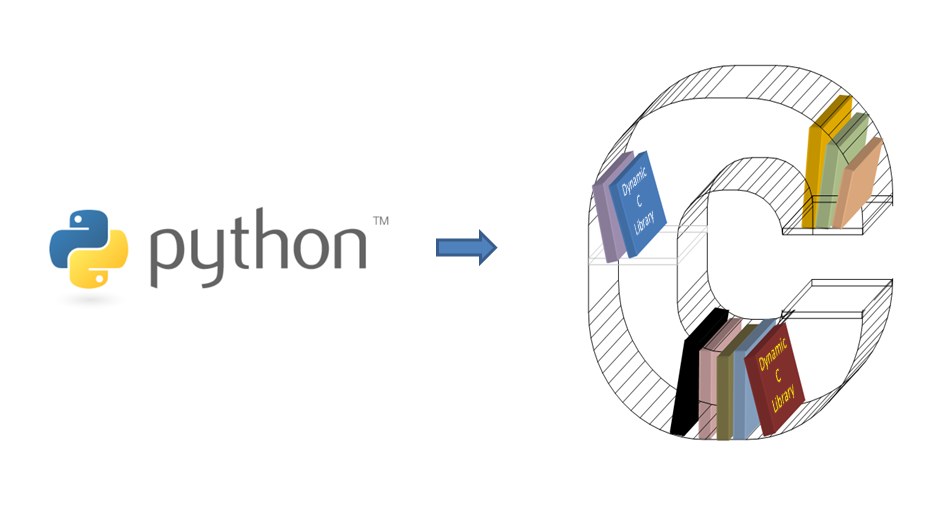# Calling C from Python

Aug 19, 2022 by Charles Beumier | 1357 views

Whatever the reason you would like to call C functions from Python, one approach is presented here and consists in creating a C dynamic library, compiled as a .so module and accessing the library thanks to the ctypes package of Python. Explanations are given step by step and stand for a Linux environment (tested with Ubuntu20.04).You have at least two reasons to call C functions when programming with Python: to call a C library not ported to Python or to call C functions that you wrote for improved performance. For performance, always check that a Python library does not exist before considering C code writing, especially if you do not have much experience with that language. C and Python differ in many ways and may hold many surprises. A brief comparison will be given in the blog to come: 'Python or C, or Python and C'.

Do not overestimate the gain one can obtain from C compared to Python. It is mainly interpretation, at the basis of Python, which slows down execution. Many Python libraries are in fact written in C and made callable from Python thanks to an interface. On the contrary, when it comes to mathematical operations handled by the Python interpreter, the gain with C is normally important.

## 0. Create a dynamic library in C

If you are familiar with C, you just need to know that you create your shared library (.so) with a command line like:

``    CC -shared -o libLIB.so file1.o file2.o ...``

where libLIB.so is the created library file and fileX.o are object codes compiled thanks to:

``    CC -fPIC -o fileX.o fileX.c``

The flag -fPIC is needed to have 'position independent code', necessary for a shared library code to run for any address it will occupy. Please see [https://cylab.be/blog/234/creating-a-dynamic-library-in-c] for more details, like a Makefile to create the library or details to use it in C.

## 1. An example of Python calling C

Please find the Python source code (file call_Fct.py) for opening a file and telling the number of characters it contains. The C dynamic library for these file operations was presented as an example in [https://cylab.be/blog/234/creating-a-dynamic-library-in-c]. The file 'file.txt' is expected to be in the current directory and contain some text.

``````# Example ('python3 call_Fct.py') that calls a C library

import ctypes as CT

# Access library
CLIB = CT.CDLL("./libFct.so")

# Function prototypes for Python (of C functions)
# For (FILE *) in C, we have not described the (long) structure (not needed)
#   Instead, we use c_void_p (pointer to void)
CLIB.FileOpen.argtypes = [ CT.c_char_p, CT.POINTER(CT.c_int) ]
CLIB.FileOpen.restype  = CT.c_void_p
CLIB.FileSize.argtypes = [ CT.c_void_p ]
CLIB.FileSize.restype  = CT.c_int

# Calling C functions
filename = "file.txt"
filenameU8 = filename.encode("utf-8") # Convert str to byte array
name_len = CT.c_int(0)

fd = CLIB.FileOpen(filenameU8, CT.byref(name_len))
print("Name length: %d" % (name_len.value))

size = CLIB.FileSize(fd)
print("File size = %d" % (size))``````

The Python program is run by:

``python3 call_Fct.py``

## 2. Import the ctypes Python library

In Python, ctypes is the library which provides C compatible data types, and allows calling functions from C (shared) dynamic libraries. It is used to wrap the C libraries so that the functions can be called in the usual pythonic way.

ctypes provides python equivalent to C types like c_int, c_bool, c_float, c_char, c_char_p, etc. New types can be created, for instance thanks to the POINTER() function and the Structure class. We give here only a couple of examples to illustrate the important step of function prototyping.

## 3. Accessing the lib in a Python program

Following the example, the library 'libFct.so' with appropriate path can be accessed through the object returned by the ctypes CDLL function ('CLIB' in our example). This object contains all the functions and globals defined in the library.

## 4. Describe the function prototypes

A C function provided in the loaded libary has to be described so that arguments and result are appropriately passed or interpreted. CLIB.fct_name.argtypes helps to specify the list of argument types and CLIB.fct_name.restype the type of the return for function fct_name.

## 5. Format the values for C calls

When passing values to the C functions, there are cases which require special attention.

For instance, the variable filename is a str in Python which has to be converted to an array of char compatible with C strings thanks to the .encode("utf-8") function.

The second argument of the call 'FileOpen', the integer 'name_len', has to be passed by reference to return a value (in this case the length of the file name) computed by 'FileOpen'. The function CT.byref(name_len) serves this purpose.

The variable 'fd' for the return of the 'FileOpen' call represents the address to a structure holding the file descriptor in the C library. Since we do not need the details of this descriptor and because creating such a structure with many fields with ctypes requires some work, we declared the return 'fd' as a pointer to void (c_void_p). This variable can be used in other functions with arguments compatible with 'fd', such as the next call CLIB.FileSize(fd).

With this small example in hand and the common types mentioned above (c_int, c_bool, c_float, c_char, ...), you are ready to deal with many situations. If you need more specific data types or tricky cases, please refer to the ctypes reference ([https://docs.python.org/3/library/ctypes.html]).

## Final words

We presented a small example calling C functions of a C dynamic library from Python. This may reveal useful when you have a library in C or if you look for speedup thanks to C programming.